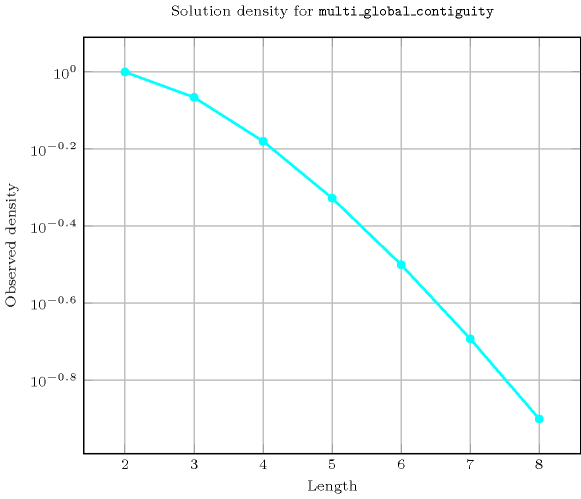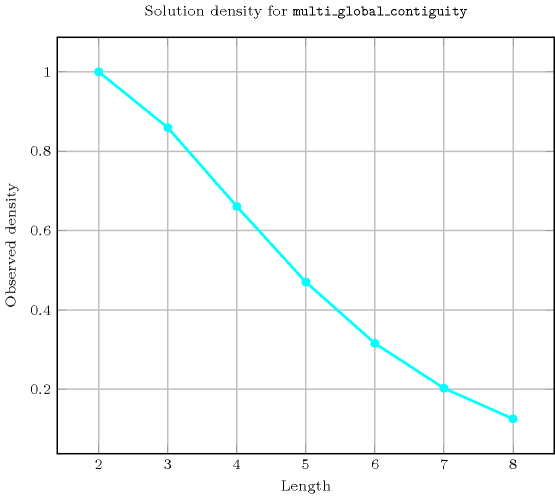## 5.267. multi_global_contiguity

Origin
Constraint

$\mathrm{𝚖𝚞𝚕𝚝𝚒}_\mathrm{𝚐𝚕𝚘𝚋𝚊𝚕}_\mathrm{𝚌𝚘𝚗𝚝𝚒𝚐𝚞𝚒𝚝𝚢}\left(\mathrm{𝚅𝙰𝚁𝙸𝙰𝙱𝙻𝙴𝚂}\right)$

Synonym

$\mathrm{𝚖𝚞𝚕𝚝𝚒}_\mathrm{𝚌𝚘𝚗𝚝𝚒𝚐𝚞𝚒𝚝𝚢}$.

Argument
 $\mathrm{𝚅𝙰𝚁𝙸𝙰𝙱𝙻𝙴𝚂}$ $\mathrm{𝚌𝚘𝚕𝚕𝚎𝚌𝚝𝚒𝚘𝚗}\left(\mathrm{𝚟𝚊𝚛}-\mathrm{𝚍𝚟𝚊𝚛}\right)$
Restrictions
 $\mathrm{𝚛𝚎𝚚𝚞𝚒𝚛𝚎𝚍}$$\left(\mathrm{𝚅𝙰𝚁𝙸𝙰𝙱𝙻𝙴𝚂},\mathrm{𝚟𝚊𝚛}\right)$ $\mathrm{𝚅𝙰𝚁𝙸𝙰𝙱𝙻𝙴𝚂}.\mathrm{𝚟𝚊𝚛}\ge 0$
Purpose

Enforce all variables of the $\mathrm{𝚅𝙰𝚁𝙸𝙰𝙱𝙻𝙴𝚂}$ collection to be assigned a value greater than or equal to 0. In addition, each value $v$ strictly greater than 0 should appear contiguously.

Example
$\left(〈0,2,2,1,1,0,0,5〉\right)$

The $\mathrm{𝚖𝚞𝚕𝚝𝚒}_\mathrm{𝚐𝚕𝚘𝚋𝚊𝚕}_\mathrm{𝚌𝚘𝚗𝚝𝚒𝚐𝚞𝚒𝚝𝚢}$ constraint holds since the sequence $02211005$ contains no more than one group of contiguous 1, no more than one group of contiguous 2, and no more than one group of contiguous 5.

Typical
$|\mathrm{𝚅𝙰𝚁𝙸𝙰𝙱𝙻𝙴𝚂}|>3$
Symmetry

Items of $\mathrm{𝚅𝙰𝚁𝙸𝙰𝙱𝙻𝙴𝚂}$ can be reversed.

Arg. properties

Contractible wrt. $\mathrm{𝚅𝙰𝚁𝙸𝙰𝙱𝙻𝙴𝚂}$.

Counting
 Length ($n$) 2 3 4 5 6 7 8 Solutions 9 55 413 3656 37147 425069 5400481

Number of solutions for $\mathrm{𝚖𝚞𝚕𝚝𝚒}_\mathrm{𝚐𝚕𝚘𝚋𝚊𝚕}_\mathrm{𝚌𝚘𝚗𝚝𝚒𝚐𝚞𝚒𝚝𝚢}$: domains $0..n$